## Introduction

The rocksalt IV–VI semiconductors have been identified by Fu et al.1,2 as a novel class of insulators—the topological crystalline insulators—which display surface states that are protected by crystalline symmetry. The topological surface states in topological crystalline insulators are to be contrasted with those in the widely investigated Z2 invariant topological insulators, which are protected by time-reversal invariance3,4. Angle-resolved photoemission spectroscopy (ARPES) experiments have obtained evidence for the surface states in Pb1−xSnxSe (ref. 5), SnTe (ref. 6) and Pb1−xSnxTe (ref. 7).

In the alloys Pb1−xSnxTe and Pb1−xSnxSe, the bulk electrons occupy four small Fermi surface (FS) pockets located at the L points in k space (inset, Fig. 1). The conduction band is predominantly derived from the cation Pb 6p orbitals, whereas the uppermost valence band is predominantly anion 4p (or 5p) orbitals (ordering similar to the atomic limit)8. As the Sn content x increases, the system undergoes gap inversion when x exceeds a critical value xc (refs 9, 10, 11, 12). In samples with xxc, gap inversion occurs when the temperature T is lowered below the gap-inversion temperature Tinv. The ARPES experiments5,6,7 confirm that the predicted topological surface states appear in the gap-inverted phase.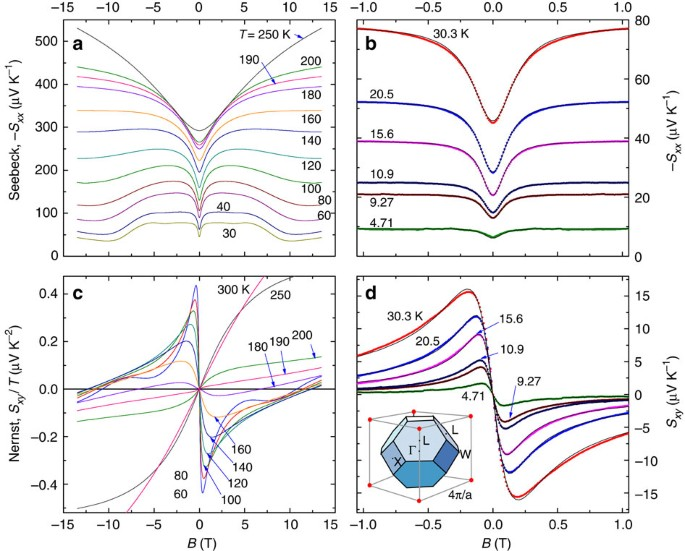Figure 1: Thermopower and Nernst effect in a topological crystalline insulator.

The new topological ideas invite a fresh look at the bulk states of the IV–VI semiconductors. To date, the gap inversion appears to have no discernible effect on transport properties (the resistivity, Hall coefficient and thermopower vary smoothly through Tinv). This is surprising given that transport probes the states at the Fermi level. Moreover, a long-standing prediction8,13 is that the bulk electrons occupy states described by the massive Dirac Hamiltonian. This assumption underlies the starting Hamiltonian of Hsieh et al.2 However, no experimental test distinguishing the massive Dirac from the Schrödinger Hamiltonian has appeared to our knowledge.

We have grown crystals of Pb1xSnxSe (x=0.23) in which the n-type carriers have high mobilities (μ=114,000 cm2 V−1 s−1 at 4 K). The low electron density (3.46 × 1017 cm−3) enables the quantum limit to be reached at 7.7 T (measurements reveal that holes are absent). In addition to resistivity, we have used both thermopower and the Nernst effect to probe the states in fields up to 34 T. Surprisingly, the Nernst signal is observed to change its sign at Tinv. To date, this appears to be the only transport or thermodynamic quantity that is strongly affected by gap inversion.

In a thermal gradient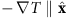and an applied magnetic field, the diffusion of carriers produces an electric field E, which is expressed as the thermopower signal Sxx=−Ex/|T| and the Nernst signal Sxy=Ey/|T|. In the semiclassical regime, the Mott relation14 simplifies Sxx and Sxy to the form (see Methods section)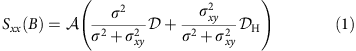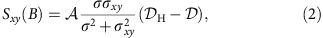where. The dependence on B appears only in the conductivity matrix elements σij(B) (for brevity, we write σσxx). The parameters =∂lnσ/∂ζ and H=∂lnσxy/∂ζ are independent of the mobility μ (ζ is the chemical potential). Equation 1 describes the crossover in Sxx from (at B=0) to H when μB>>1. Correspondingly, Sxy increases linearly from 0 to peak at the value 1/2(H−) at B=1/μ before falling as 1/B when μB>>1. For n-type carriers, both and σxy are negative, and Sxx<0. From equation 2, the Nernst signal Sxy is positive if H> (we discuss the sign convention in the Methods section). In terms of the exponents β and βH defined by σ(E)~Eβ and σxy~${E}^{{\beta }_{\text{H}}}$, we have =β/EF and H=βH/EF.

Even for one-band systems, equations 1 and 2 have not received much experimental attention, possibly because real materials having only a single band of carriers with a low density (and high mobility) are rare. The analysis of Sij is complicated by the extreme anisotropy of the FS pockets in many semi-metals. For recent Nernst measurements on Bi and Bi2Se3, see refs 15, 16. For results on Sij in graphene, see refs 17, 18, 19. The angular variation of the SdH period in Bi is investigated in refs 20, 21. In the annealed crystals of Pb1−xSnxSe (with x~xc) investigated here, our measurements reveal only n-type carriers (holes are absent). The FS pockets are very small (the quantum limit is reached at 7.7 T) and nearly isotropic (as shown by field-tilt measurements). We find that equations 1 and 2 provide a very good fit to Sxx and Sxy in the semiclassical regime.

Here we provide evidence that the bulk electronic states at the L points are in fact massive Dirac states. A characteristic feature of the massive Dirac spectrum is that in a magnetic field, the lowest Landau level (LL) is non-degenerate with respect to the spin degrees, whereas all higher LLs are doubly degenerate. Knowing the carrier density, we show that the field at which the chemical potential jumps to the lowest LL accurately determines its spin degeneracy to be 1. This confirms the starting assumption of ref. 2. The unusual thermoelectric response is also investigated deep in the quantum limit. In addition, we show that the sign of the Nernst signal is anomalous (relative to standard Boltzmann theory) within the gap-inverted phase.

## Results

### Semiclassical regime

Figure 1a-1b plots curves of the thermopower Sxx versus B for selected T. From 250 to 160 K, the dominant feature is the rapid increase in weak B followed by saturation to a B-independent plateau at large B. As noted, the Nernst signal (shown as Sxy/T in Fig. 1c) changes from positive to negative as T is decreased below 180 K (identified with Tinv).

As shown in Fig. 1b, the curves of Sxx versus B fit very well to equation 1 in the semiclassical regime (|B|<1T). Likewise, below 100 K the curves of Sxy also fit well to equation 2 up to an overall sign (Fig. 1d). Although the fit parameters (μ, , H) for Sxx are independent of those for Sxy, we find that they agree with each other (at the level of ±2%) below 60 K (see Methods section). At each T, the two curves, Sxx(B) and Sxy(B), are described by just three parameters. This provides a potent self-consistency check of equations 1 and 2. As a further test, we have also fitted the measured conductivity tensor σij(B) and obtained similar values for μ below 100 K (Methods). By and large, the close fits to both tensors Sij and σij demonstrate that we have one band of carriers below 100 K.

The semiclassical expressions are no longer valid when quantum oscillations appear at higher B. In particular, the giant step at 7.7 T in the curves at 30 and 40 K (Fig. 1a) is a relic of the quantum regime that remains resolvable up to 100 K. The step has a key role in the discussion later. In the opposite extreme above 100 K, the two sets of fit parameters begin to deviate. The disagreement is especially acute near 180 K, where Sxy changes sign. We reason that the one-band assumption breaks down because of strong thermal activation of holes as the gap closes and re-opens across Tinv. The evidence comes from the T dependence of the Hall density nH=B/yx (solid circles in Fig. 2a). Whereas nH is nearly T-independent below 80 K, in agreement with the one-band model, it deviates upwards above 180 K. Thermal activation of a large population of holes leads to partial cancellation of the Hall E-field and a reduction in |ρyx|.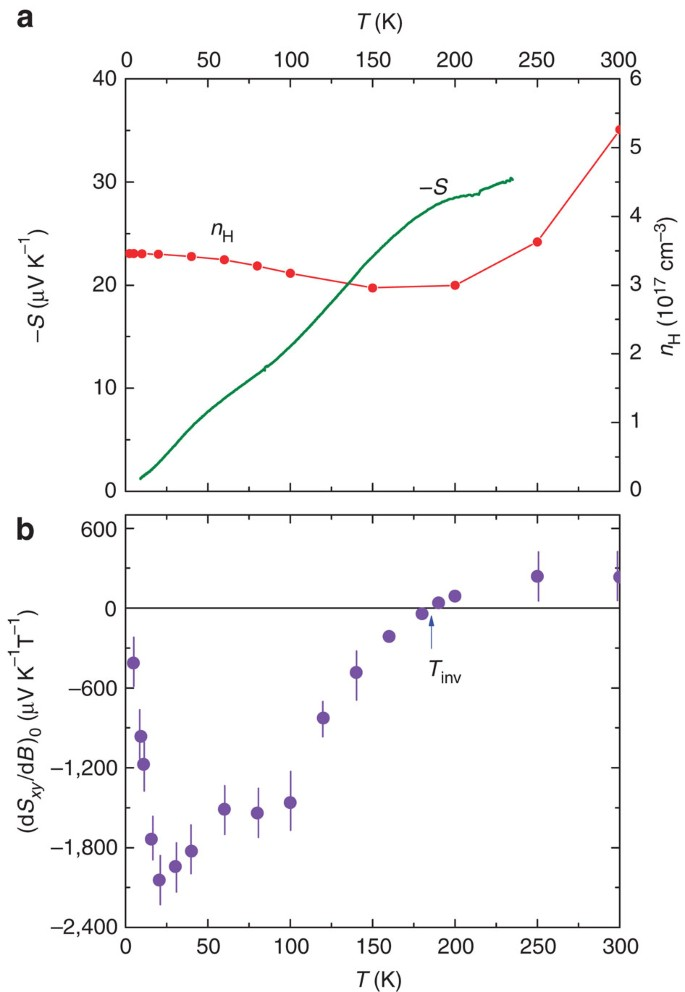Figure 2: Temperature dependence of Hall density, thermopower and Nernst slope.

In Fig. 2a, we have also plotted the zero-H thermopower S≡Sxx(0) to bring out its nominally T-linear variation below 100 K (bold curve). The large value of the slope S(T)/T=1.41 μV K−2 implies an unusually small EF.

As discussed, the Nernst signal changes sign at Tinv=180 K. The T dependence of its initial slope dSxy/dB (B→0) is displayed in Fig. 2b. From the fits to equations 1 and 2, we may address the interesting question whether the sign anomaly occurs in the gap-inverted phase (T<Tinv) or in the uninverted phase. On both sides of Tinv, the fits of Sxx imply H> (that is, |Sxx| always increases as μB goes from 0 to values >>1). As Sxy~(H−), we should observe a positive Sxy. Hence, the sign anomaly occurs in the gap-inverted phase (in Fig. 1d, we multiplied the curves by an overall minus sign). The sign of the Nernst signal below Tinv disagrees with that inferred from equations 1 and 2, despite the close fit. Further discussion of the sign anomaly is given below (see Discussion section). However, we note that the sign of Sxy is independent of the carrier sign. As seen in Fig. 2a, both S and nH vary smoothly through Tinv without a sign change.

### Quantum oscillations

As shown in Fig. 3, oscillations in Sxx and Sxy grow rapidly below 60 K to dominate the weak-B semiclassical profile. The most prominent feature in Sxx is the large step decrease at the field B1=7.7 T (at which the chemical potential ζ jumps from the N=1 LL to the N=0 LL). In the Nernst curves, plotted as Sxy/T in Fig. 3b, the quantum oscillations are more sharply resolved. As Sxy is the off-diagonal term of the tensor Sij, its maxima (or minima) are shifted by 1/4 period relative to the extrema of the diagonal Sxx (analogous to the shift of σxy relative to σ). This shift is confirmed in Fig. 4a, which plots the traces of Sxx and Sxy versus 1/B. For the analysis below, we ignore the weak spin splitting, which is resolved in the N=1 LL (and barely in N=2).Figure 3: Quantum oscillations in the thermopower and Nernst signal of PbSnSe.

Figure 4b shows the index plot of 1/Bn (inferred from the maxima in |Sxx| and Sxy) plotted versus the integers n. From the slope of the line, we derive the FS section SF=5.95 T=5.67 × 1016 m−2. Assuming a circular cross-section, we have kF=0.0134 Å−1. The electron concentration per FS pocket is then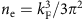=8.2 × 1016 cm−3. As there are four pockets, the total carrier density is 4ne=3.28 × 1017 cm−3, in good agreement with the Hall density nH at 4 K (3.46 × 1017 cm−3).

Using sample 2, we have tracked the variation of the SdH period versus the tilt angle θ of B. Figure 5a plots the fields B1 and B2 versus θ (B is rotated in the yz plane). Here, B1 and B2 are the fields at which ζ jumps from N=1→0 and from N=2→1, respectively. To our resolution, the SdH period is nearly isotropic. The fields B1 and B2 are also independent of tilt angle when B is rotated in the xy plane. This justifies treating the FS pockets as nominally spherical.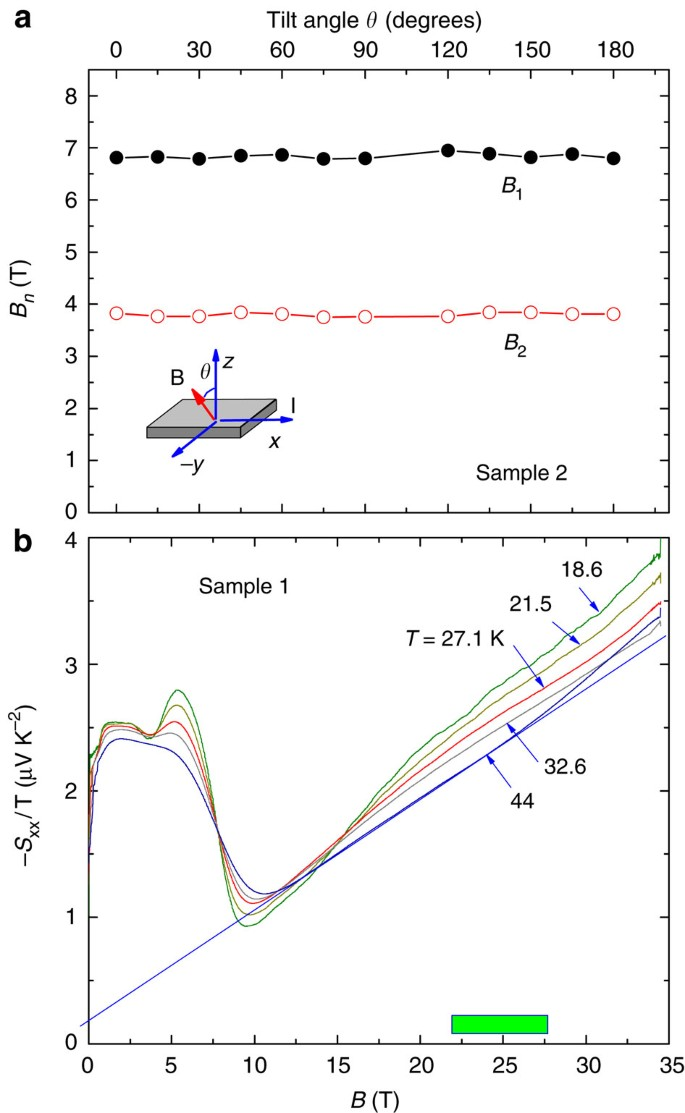Figure 5: Dependence of SdH period on field-tilt angle and high-field thermopower.

### The N=0 Landau level

We next address the question whether the bulk states in the inverted phase are Dirac fermions or Schrödinger electrons. The two cases differ by a distinctive feature in their LL spectrum that is robust against small perturbations. In the quantum limit, the massive Dirac Hamiltonian exhibits an interesting twofold difference in degeneracy between the N=0 and N=1 levels. Wolff22 considered a three-dimensional (3D) massive Dirac Hamiltonian with spin–orbit interaction but no Zeeman energy term. More recently, Serajedh et al.23 included the Zeeman energy term as well as a Rashba term in the massive two-dimensional (2D) Dirac Hamiltonian. Other 3D massive Dirac cases are discussed by Bernevig and Hughes24. All these authors find that N=0 LL is non-degenerate with respect to spin degrees, whereas the LLs with N≠0 are doubly spin degenerate (we discuss in Methods section a pedagogical example, which shows that this anomaly is related to the conservation of states). By contrast, for the Schrödinger case, all LLs are doubly degenerate.

In Pb1−xSnxSe, the ability to measure accurately both ne and the ‘jump’ field B1 provides a crisp confirmation of this prediction.

The energy of the Nth LL is, where mD is the Dirac mass and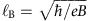the magnetic length24. At B1, EF lies just below the bottom of the N=1 LL so that all the electrons are accomodated in the N=0 LL. Integrating the density of states (DOS) for one spin polarization in the N=0 LL from mDv2 to EF, we find (see Methods section)Ignoring the small spin splitting, we equate B1 with 7.7 T. Equation 3 then gives ne↑=9.0 × 1016 cm−3, which agrees within 10% with the measured ne (the agreement is improved if we correct for spin splitting). All the electrons are accomodated by an N=0 LL that is non-degenerate, in agreement with the prediction for massive Dirac states22,23,24, but disagreeing with the Schrödinger case by a factor of 2. As the singular spin degeneracy of the N=0 LL cannot be converted to a double degeneracy, the experiment uncovers a topological feature of the bulk states that is robust. As predicted in refs 22, 23, 24, the N=0 LL has only one spin state (0,+); the spin-down partner (0,−) is absent.

To check this further, we extended measurements of Sxx to 34 T to search for the transition from the sublevel (0,−) to (0,+) (which should occur if N=0 LL were doubly degenerate). From extrapolation of the spin split N=1 and N=2 LLs, we estimate that the transition (0,−)→(0,+) should appear in the interval 22–28 T. As shown in Fig. 5b, the measured curves show no evidence for this transition to fields up to 34 T.

Finally, we note an interesting thermopower feature in the quantum limit. At fields above B1, Sxx displays a B-linear profile that extends to 34 T (Fig. 5). The B-linear behaviour is most evident in the curve at 44 K. As T is decreased to 18.6 K, we resolve a slight downwards deviation from the linear profile in the field interval 10–20 T. The B-linear profile appears to be a characteristic property of massive Dirac fermions in the quantum limit. We discuss below a heuristic, semiclassical approach that reproduces the observed profile.

## Discussion

We summarize the electronic parameters inferred from our experiment and relate them to ARPES measurements.

As noted, the FS section derived from the index plot (Fig. 4b) corresponds to a total electron density 4ne=3.28 × 1017 cm−3, in good agreement with the Hall density nH at 4 K (3.46 × 1017 cm−3).

We may estimate EF from the slope of the thermopower S(T)/T=1.41 μV K−2. Using the Mott expression S(T)=(π2/3)(kB/e)(kBT/EF)β, we find for the Fermi energy EF=17.0 β meV. For the massive Dirac dispersion, we have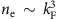, which implies that β has the minimum value 3 (if the mobility increases with E, β is larger). Using the lower bound, β=3, S/T gives EF=51 meV.

These numbers may be compared with ARPES measurements. We estimate the Fermi velocity from the expression v~EF/ħkF (valid when EFmDv2 with mD the Dirac mass). Using our values of EF and kF, we find v=5.74 × 105 m s−1 as the lower bound. Although ARPES experiment cannot resolve v in the conduction band, the ARPES estimate5 for the hole band velocity is 5.6 × 105 m s−1, in good agreement with our lower bound. It is likely to be that the conduction band has a higher velocity (which would then require β>3).

One of our findings is that gap inversion changes the sign of the Nernst signal. As the energies of states involved in gap inversion are very small, the resulting dispersion can be hard to resolve by ARPES measurement. Transport quantities would appear to be more sensitive to these changes. As noted, however, most transport quantities are either unaffected or only mildly perturbed. The Hall effect and thermopower are unchanged in sign across Tinv (Fig. 2a). Although nH shows a gradual increase, this is largely attributed to thermal activation of holes across a reduced gap for T>Tinv. Hence, the dramatic sign change observed in Sxy stands out prominently; its qualitative nature may provide a vital clue.

It has long been known10 that in the lead rocksalt IV–VI semiconductors, the energy gap Eg undergoes inversion as the Sn content x increases from 0. Moreover, within a narrow range of x, gap inversion is also driven by cooling a sample (the critical temperature is x dependent within this interval). Strauss10 performed early optical transmission measurements of Eg in a series of single-crystal films of Pb1−xSnxSe, with x ranging from 0 to 0.35. For x=0.25, he reported that Eg closes at 195 K. A slight interpolation of his data shows that at our doping x=0.23, Eg should vanish at 179 K, remarkably close to our Tinv=180 K. The recent ARPES measurements of Dziawa et al.5 is consistent with Eg closing between 100 and 200 K. Given the ARPES resolution, these results are all consistent with our inference that our Tinv corresponds to the gap inversion temperature. Hence, we reason that the Nernst signal changes sign either at, or very close to, the gap inversion temperature. The inverted sign of Sxy below 180 K in Fig. 1c,d occurs in the gap-inverted phase. We refrain from making the larger claim that this is also the topological transition because we are unable to resolve the surface states in our experiments.

The fits of Sxy to equation 2 (Fig. 1d) shows that the curves below 100 K are well described by the Boltzmann–Mott expression assuming a single band of carriers, but there is an overall sign disagreement. Despite the sign problem, the analysis singles out the physical factors that fix the sign and delineates the scope of the problem. For example, reversing the sign of both β and βH inverts the sign of Sxy, but also that of Sxx. Alternately, one might try reversing the signs of β and βH, and e simultaneously. This will invert the sign of Sxy but leave Sxx unchanged. However, ρyx is forced to change sign.

The analysis assumes that in the gap-inverted phase, the FS is simply connected. This may not be valid. Gap inverion may lead to the existence of a small pocket surrounded by a larger FS sheet (topologically similar to the FS of the ‘giant Rashba’ material BiTeI25). As the small pocket dominates the thermoelectric response, the Nernst effect may be detecting this novel situation. These issues will be left for future experiments.

We may attempt to understand the striking B-linear profile of Sxx/T in Fig. 5 using a semiclassical approach. In N=0 LL, the long-lived quasiparticles complete a large number of cyclotron orbits between scattering events (for example, from μB~220, we estimate this number is ~35 at 20 T). The scattering results in the drift of the orbit centres X in a direction transverse to the applied −T. Ignoring the fast cyclotron motion, we may apply the Boltzmann equation to X. The thermopower is then given by the high-B limit of equation 1, Sxx(T,H)→βH′/EF, where EF is now measured from the bottom of N=0 LL and βH′ differs from the weak-field βH. In this picture, the B dependence of Sxx arises solely from how EF changes with B.

For B>B1, only N=0 LL is occupied. From equation 14 (Methods), we have the relation between EF, ne and B, viz.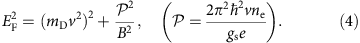In the limit EFmDv2, we obtain the relation EF~1/B. This immediately implies that Sxx/T increases linearly with B as observed. Setting gs=1, we derive from equation 4 the rate of increase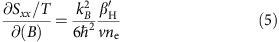Repeating this calculation for the Schrödinger case, we get instead Sxx/T~B2.

From Fig. 5, the thermopower slope ∂(Sxx/T)/∂(B)=8.71 × 10−8 V K−2 T−1. Using the above values of v and ne in equation 5, we find (∂Sxx/T)/∂(B)=6.1 βH × 10−8 V K−2 T−1. The value of βH is not known. Comparison of the calculated slope with experiment suggests βH~1.5. Hence, this back-of-the-envelop estimate can account for the rate at which Sxx/T increases with B.

## Methods

### Semiclassical fits to Sxx and Sxy

In the presence of a magnetic field B, an electric field E and a temperature gradient −T (in an infinite medium), the total current density is given by14 J=σ·E+α·(−T). Here, σij is the conductivity tensor and αij is the thermoelectric tensor. Setting J=0 (for a finite sample) and solving for E, we have E=−ρ·α·(−T), with ρ=σ−1 the resistivity tensor.

In the geometry with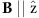and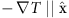, the components of the E-field (for an isotropic system) are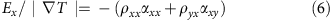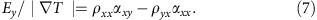The thermoelectric tensor Sij is given by Ei=SijjT (Sxx>0 for hole carriers and Sxy>0 if Ey>0 when Hz>0).

The Mott relation14,where kB is Boltzmann’s constant, e is the elemental charge and ζ the chemical potential, has been shown to hold under general conditions, for example, in the quantum Hall Effect (QHE)26,27. Using equation 8, equations 6 and 7 reduce to equations 1 and 2, respectively.

The fits of Sij to these equations displayed in Fig. 1d were carried out using the one-band, Boltzmann–Drude expressions for the conductivity tensor, viz.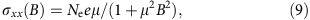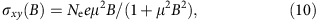where the total carrier density Ne is 4ne (ne is the density in each of the FS pocket at the L points).

In the geometry withand, we define the sign of the Nernst signal to be that of the y component of the E-field Ey. More generally, if EN is the E-field produced by the Nernst effect, the sign of the Nersnt signal is that of the triple product EN·B × (−T). This agrees with the old convention based on ‘Amperean current’28 and with the one adopted for vortex flow in superconductors29.

At each T, we have fitted the measured curves of Sxx and Sxy versus B to equations 1 and 2 using equations 9 and 10 for the conductivity tensor. The separate fits of Sxx and Sxy yield two sets of the parameters μ, and H, which are displayed in Fig. 6 (solid triangles and open circles, respectively). The three-parameter fit places strong constraints on the curves of Sxx and Sxy. Disagreement between the two sets signals that the one-band model is inadequate.

Below 100 K, the two sets agree well, whereas closer to Tinv they begin to deviate. The reason is that equation 2 cannot account for the change of sign in the Nernst signal given the relative magnitudes of and H fixed by the curves of Sxx. Above 200 K, the two sets are inconsistent because thermal excitations of holes across the small band gap is important at elevated T and the one-band assumption becomes inadequate. This is evident in the onset above 200 K of significant T dependence in the Hall density nH (see Fig. 2a).

We remark that Sxx=VxT is directly obtained from the observed voltage difference Vx and the temperature difference δT between longitudinal electrical contacts (their spatial separation Lx is immaterial). However, for the Nernst signal, we have Sxy=(VyT)(Lx/Ly), where Ly is the spatial separation between the transverse contacts. Hence, the aspect ratio Ly/Lx is needed to convert the observed Nernst voltage Vy to Sxy. The ratio Ly/Lx is measured to be 4±0.4. The fits are improved significantly if this value is refined to 4.20, which we adopt for the curves at all T.

Fits to equation 9 and 10 of the conductivity tensor measured in the same sample are shown in Fig. 7 for weak B at selected T from 5 to 150 K. The fits yield values of the mobility μ similar to those shown in Fig. 6a. The inferred carrier density Ne is also similar to the measured Hall density nH.

### Indexing the quantum oscillations

For the 3D systems, one identifies the index field Bn as the field at which the DOS displays a sharp maximum (diverging as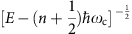in the absence of disorder). From the quantization rule for areas in k-space, Bn is related to the FS cross-section F as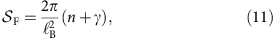whereand γ (the Onsager phase) isfor Schödinger electrons. The plot in Fig. 3b follows equation 11. From its slope, we obtain F. The intercept γ is close to zero in Fig. 3b. We will discuss γ elsewhere.

We note that, in the 2D systems in the QHE regime, the index field is the field at which the chemical potential ζ falls between adjacent LLs, where the DOS vanishes, and the Hall conductance displays a plateau. The difference between 2D and 3D systems arises because the integer n counts the number of edge states in the QHE case, whereas n indexes the DOS peaks in the 3D case. One needs to keep this in mind in interpreting γ.

We have verified that the slope in Fig. 4b is insensitive to the tilt angle θ of B relative to the crystalline axes. As shown in Fig. 5a, the SdH period is virtually independent of θ within the experimental uncertainties, consistent with negligible anisotropy in the small FS pockets. The good agreement between F and nH (Hall density) at 5 K is also evidence for a negligible anisotropy.

### Spin degeneracy in N=0 LL

Knowledge of the field B1 (the transition from N=1 to the N=0 LL) and the electron density per valley ne suffices to determine the spin degeneracy of the N=0 LL.

For the 3D Dirac case24, the energy in the Nth LL is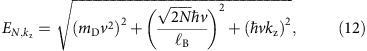with mD the Dirac mass, kz the component of k along B andthe magnetic length.

For N=0 LL, we solve for kz(E)where E00=mDv2.

Let us assume that only N=0 LL is occupied. To obtain the relation linking EF, B and ne, we integrate the 3D DOS (E)dE=(gLgs/π)dkz, with gs the spin degeneracy and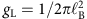the 2D LL degeneracy per spin. Using equation 13, we have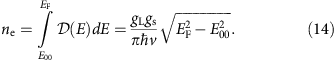This equation is valid until B is reduced to the jump field B1, whereafter electrons enter the N=1 LL. At the jump field, EF lies just below the bottom of N=1 LL, that is,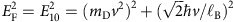. Using this in equation 14, we have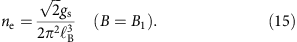In relation to equation 3, we showed that equation 15 gives a value equal (within 10%) to the total electron density per valley if gs=1, that is, when B>B1, all the electrons can be accomodated by the N=0 LL with only one spin polarization. This is direct evidence for the non-degeneracy of the N=0 LL.

Interestingly, equation 15 is identical for the isotropic Schrödinger case, for which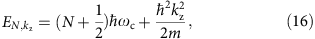where ωc=eB/m and m is the mass. However, for N=0 LL of the Schrödinger spectrum, we must have gs=2, so it can be excluded.

### A simple example of massive Dirac spectrum

An example illustrating the non-degeneracy of N=0 LL is the spinless fermion on the 2D hexagonal lattice (valley degeneracy replaces spin degeneracy in this example). The sublattices A and B have distinct on-site energies εA and εB as in BN. The Dirac cones remain centred at the inequivalent ‘valleys’ K and K′ in k-space (inset, Fig. 8). Both valleys acquire a mass gap.Figure 8: The massive Dirac spectrum in the 2D hexagonal lattice.

For states close to the valley at K, the 2D massive Dirac Hamiltonian isin the basis (1,0)T (pseudospin up) and (0,1)T (pseudospin down), where k is measured from K and m>0 represents the gap parameter proportional to εAεB (we set the velocity v to 1). In a field B, we replace k by π=ke A with the vector gauge A=(0,Bx,0). Introducing the operatorswith π±=πx±y, and eigenstates |N› satisfying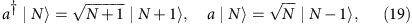we diagonalize the Hamiltonian to get eigenenergies EN given by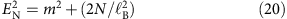(for brevity, we will write E for EN).

For positive E, the (unrenormalized) two-spinor eigenstates are (for N=0,1,)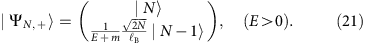For the negative energy states, the corresponding eigenvectors are (N=1,2,)Setting N=0 in equation 21, we find that the positive-energy state |Ψ0,+›=(|0›,0)T (pseudospin up). For E<0, however, the lower entry in equation 22 is non-determinate (0/0). This implies that the state N=0 does not exist for E<0. Thus, for the valley at K, there is only one LL with N=0. It has positive energy E0=|m|; the corresponding LL at −|m| is absent (the spectrum of K is sketched as curve 1 in Fig. 8).

Repeating the calculation for K′, we find the opposite situation (the Hamiltonian is the conjugate of equation 17). Now N=0 LL has energy E0=−|m|, but N=0 LL is absent in the positive spectrum (the spectrum of K′ is the curve 2 in Fig. 8).

A transport experiment detects the sum of the two spectra (curves of K+K′ at different B are collectively labelled as 3 in Fig. 8). In the total spectrum, the two N=0 LLs are non-degenerate, whereas all LLs with N≠0 have a valley degeneracy of 2. The difference simply reflects the conservation of states. In the limit m→0, we recover the spectrum of graphene. If, at finite m, each of the N=0 LLs had a valley degeneracy of 2, we would end up with an N=0 LL in graphene with fourfold valley degeneracy.

The authors in refs 22, 23, 24 and others have shown that the non-degeneracy of the N=0 LL also holds in massive Dirac systems even when a Rashba term and a Zeeman energy term are included.